Understanding A Recurrent Neural Network For Image Generation by@shugert

# Understanding A Recurrent Neural Network For Image Generation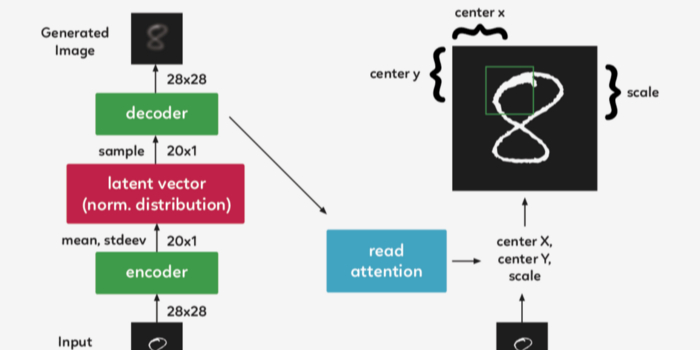The purpose of this post is to implement and understand Google Deepmind’s paper DRAW: A Recurrent Neural Network For Image Generation. The code is based on the work of Eric Jang, who in his original code was able to achieve the implementation in only 158 lines of Python code.

## Let’s begin by explaining what does DRAW stands for…

Deep Recurrent Attentive Writer (DRAW) is a neural network architecture for image generation. DRAW networks combine a novel spatial attention mechanism that mimics the foveation of the human eye, with a sequential variational auto-encoding framework that allows for the iterative construction of complex images.

The system substantially improves on the state of the art for generative models on MNIST, and, when trained on the Street View House Numbers dataset, it generates images that cannot be distinguished from real data with the naked eye.

The core of the DRAW architecture is a pair of recurrent neural networks: an encoder network that compresses the real images presented during training, and a decoder that reconstitutes images after receiving codes. The combined system is trained end-to-end with stochastic gradient descent, where the loss function is a variational upper bound on the log-likelihood of the data.

## DRAW Architecture

DRAW Network is similar to other variational auto-encoders, it contains an encoder network that determines a distribution over latent codes that capture salient information about the input data and a decoder network receives samples from the code distribution and uses them to condition its own distribution over images.

## 3 Key Differences Between DRAW and Auto-Encoders

Both, the encoder and decoder are recurrent networks in DRAW.Decoder’s output are added successively to the distribution in order to generate the data, instead of generating this the distribution in single steps.A dynamically updated attention mechanism is used to restrict both the input region observed by the encoder, and the output region modified by the decoder. In simple terms, the network decides at each time-step “where to read” and “where to write” as well as “what to write”.Left: Conventional Variational Auto-Encoder.
During generation, a sample z is drawn from a prior P(z) and passed through the feedforward decoder network to compute the probability of the input P(x|z) given the sample.

During inference the input x is passed to the encoder network, producing an approximate posterior Q(z|x) over latent variables. During training, z is sampled from Q(z|x) and then used to compute the total description length KL ( Q (Z|x)∣∣ P(Z)−log(P(x|z)), which is minimized with stochastic gradient descent.

Right: DRAW Network.
At each time-step a sample z_t from the prior P(z_t) is passed to the recurrent decoder network, which then modifies part of the canvas matrix. The final canvas matrix cT is used to compute P(x|z_1:T).

During inference the input is read at every time-step and the result is passed to the encoder RNN. The RNNs at the previous time-step specify where to read. The output of the encoder RNN is used to compute the approximate posterior over the latent variables at that time-step.

## Loss Function

The final canvas matrix cT is used to parametrize a model D(X | cT) of the input data. If the input is binary, the natural choice for D is a Bernoulli distribution with means given by σ(cT). The reconstruction loss Lx is defined as the negative log probability of x under D: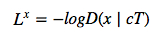The latent loss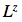for a sequence of latent distributionsis defined as the summed Kullback-Leibler divergence of some latent prior P(Z_t) from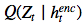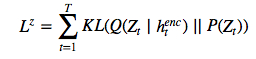Note that this loss depends upon the latent samples z_t drawn from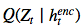which depend in turn on the input x. If the latent distribution is a diagonal Gaussian with μt, σt where: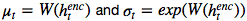a simple choice for P(Z_t) is a standard Gaussian with mean zero and standard deviation one, in which case the equation becomes: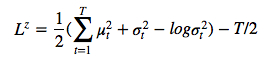The total loss L for the network is the expectation of the sum of the reconstruction and latent losses: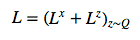Which we optimize using a single sample of z for each stochastic gradient descent step.

L^z can be interpreted as the number of nats required to transmit the latent sample sequence z_1:T to the decoder from the prior, and (if x is discrete) L^x is the number of nats required for the decoder to reconstruct x given z_1:T. The total loss is therefore equivalent to the expected compression of the data by the decoder and prior.

## Improving Images

As Eric Jang mentions on his post, it’s easier to ask our neural network to merely “improve the image” rather than “finish the image in one shot”. Human artists work by iterating on their canvas, and infer from their drawing what to fix and what to paint next.

Improving an image or progressive refinement is simply breaking up our joint distribution P(C) over and over again, resulting in a chain of latent variables C1,C2,…CT−1 to a new observed variable distribution P(CT).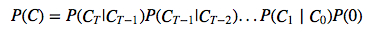The trick is to sample from the iterative refinement distribution P(Ct|Ct−1)several times rather than straight-up sampling from P(C).

In the DRAW model, P(Ct|Ct−1) is the same distribution for all t, so we can compactly represent this as the following recurrence relation (if not, then we have a Markov Chain instead of a recurrent network)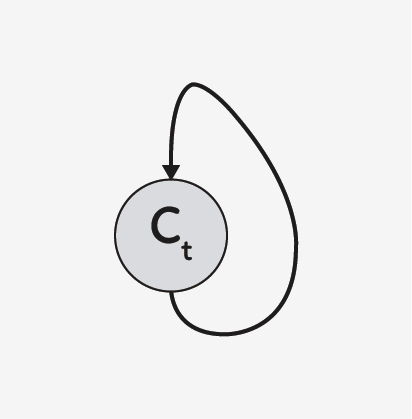## The DRAW model applied

Imagine you are trying to encode an image of the number 8. Every handwritten number is drawn differently, while some portions may be thicker others can be longer. Without attention, the encoder would be forced to try and capture all these small variations at the same time.

But…what about if the encoder could choose a small crop of the image on every frame and examine each portion of the number one at a time? That would make the work more easy, right?

The same logic applies for generating the number. The attention unit will determine where to draw the next portion of the number 8 -or any other-, while the latent vector passed will determine if the decoder generates a thicker area or a thinner area.

Basically, if we think of the latent code in a VAE (variational auto-encoder)as a vector that represents the entire image, the latent codes in DRAW can be thought of as vectors that represent a pen stroke. Eventually, a sequence of these vectors creates a recreation of the original image.

## Ok, But how does it really work?

In a recurrent VAE model, the encoder takes in the entire input image at every single timestep. In DRAW we need to focus in the attention gate between the two of them, so the encoder only receives the portion of our image that the network deems is important at that timestep. That first attention gate is called the “read” attention.

The “read” attention consists in two parts:

Choosing the important portionCropping the image and forget about other parts

## Choosing the important portion of an image

In order to determine which part of the image to focus on, we need some sort of observation to make a decision based on. In DRAW, we use the previous timestep’s decoder hidden state. Using a simple fully-connected layer, we can map the hidden state to three parameters that represent our square crop: center x, center y, and the scale.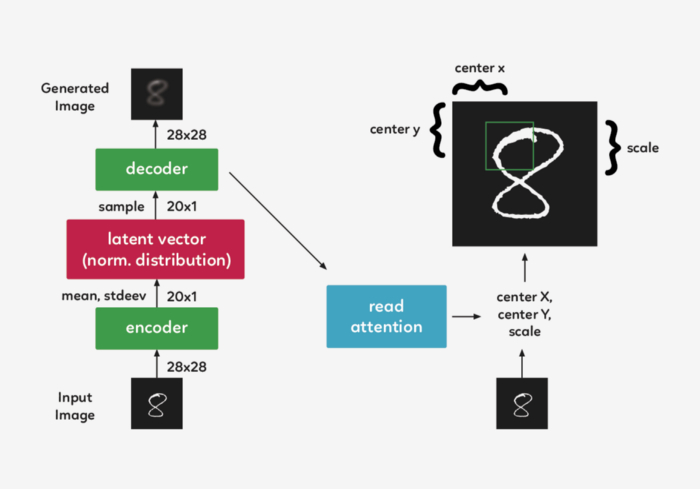## Cropping the image

Now, instead of encoding the entire image, we crop it so only a small part of the image is encoded. This code is then passed through the system, and decoded back into a small patch.

We now arrive to the second part of our attention gate, the “write”attention, which have the same setup as the “read” section, except that the “write” attention gate uses the current decoder instead of the previous timestep’s decoder.## Wait…is that really done in practice?

While describing the attention mechanism as a crop makes sense intuitively, in practice, a different method is used. The model structure described above is still accurate, but a matrix of gaussian filters instead of a crop is used.

In DRAW, we take an array of gaussian filters, each with their centers spaced apart evenly.

## Show me the money…or the code instead

We will use Eric Jang’s code as a base but we will clean it up a bit and comment it in order to make it more easy to understand

``````# first we import our libraries
import tensorflow as tf
from tensorflow.examples.tutorials import mnist
from tensorflow.examples.tutorials.mnist import input_data
import numpy as np
import scipy.misc
import os``````

Eric provide us with some great functions that will help us build our “read” and “write” attention gates as well as a function to filter the initial state that we will use below, but first, we will need add new functions that will allow us to create a dense layer as well as merge the images and save them into our local machine for our updated code.

``````# fully-conected layer
def dense(x, inputFeatures, outputFeatures, scope=None, with_w=False):
with tf.variable_scope(scope or "Linear"):
matrix = tf.get_variable("Matrix", [inputFeatures, outputFeatures], tf.float32, tf.random_normal_initializer(stddev=0.02))
bias = tf.get_variable("bias", [outputFeatures], initializer=tf.constant_initializer(0.0))
if with_w:
return tf.matmul(x, matrix) + bias, matrix, bias
else:
return tf.matmul(x, matrix) + bias

# merge images
def merge(images, size):
h, w = images.shape, images.shape
img = np.zeros((h * size, w * size))
for idx, image in enumerate(images):
i = idx % size
j = idx / size
img[j*h:j*h+h, i*w:i*w+w] = image
return img

# save image on local machine
def ims(name, img):
# print img[:10][:10]
scipy.misc.toimage(img, cmin=0, cmax=1).save(name)``````

Let’s now put the code all together for the sake of completion.

``````# DRAW implementation
class draw_model():
def __init__(self):

print "------------------------------------"
print "MNIST Dataset Succesufully Imported"
print "------------------------------------"
self.n_samples = self.mnist.train.num_examples

# We set up the model parameters
# ------------------------------
# image width,height
self.img_size = 28
self.attention_n = 5
# number of hidden units / output size in LSTM
self.n_hidden = 256
# QSampler output size
self.n_z = 10
# MNIST generation sequence length
self.sequence_length = 10
# training minibatch size
self.batch_size = 64
# workaround for variable_scope(reuse=True)
self.share_parameters = False

# Build our model
self.images = tf.placeholder(tf.float32, [None, 784]) # input (batch_size * img_size)
self.e = tf.random_normal((self.batch_size, self.n_z), mean=0, stddev=1) # Qsampler noise
self.lstm_enc = tf.nn.rnn_cell.LSTMCell(self.n_hidden, state_is_tuple=True) # encoder Op
self.lstm_dec = tf.nn.rnn_cell.LSTMCell(self.n_hidden, state_is_tuple=True) # decoder Op

# Define our state variables
self.cs =  * self.sequence_length # sequence of canvases
self.mu, self.logsigma, self.sigma =  * self.sequence_length,  * self.sequence_length,  * self.sequence_length

# Initial states
h_dec_prev = tf.zeros((self.batch_size, self.n_hidden))
enc_state = self.lstm_enc.zero_state(self.batch_size, tf.float32)
dec_state = self.lstm_dec.zero_state(self.batch_size, tf.float32)

# Construct the unrolled computational graph
x = self.images
for t in range(self.sequence_length):
# error image + original image
c_prev = tf.zeros((self.batch_size, self.img_size**2)) if t == 0 else self.cs[t-1]
x_hat = x - tf.sigmoid(c_prev)
#sanity check
print r.get_shape()
# encode to guass distribution
self.mu[t], self.logsigma[t], self.sigma[t], enc_state = self.encode(enc_state, tf.concat(1, [r, h_dec_prev]))
# sample from the distribution to get z
z = self.sampleQ(self.mu[t],self.sigma[t])
#sanity check
print z.get_shape()
# retrieve the hidden layer of RNN
h_dec, dec_state = self.decode_layer(dec_state, z)
#sanity check
print h_dec.get_shape()
# map from hidden layer
self.cs[t] = c_prev + self.write_basic(h_dec)
h_dec_prev = h_dec
self.share_parameters = True # from now on, share variables

# Loss function
self.generated_images = tf.nn.sigmoid(self.cs[-1])
self.generation_loss = tf.reduce_mean(-tf.reduce_sum(self.images * tf.log(1e-10 + self.generated_images) + (1-self.images) * tf.log(1e-10 + 1 - self.generated_images),1))

kl_terms = *self.sequence_length
for t in xrange(self.sequence_length):
mu2 = tf.square(self.mu[t])
sigma2 = tf.square(self.sigma[t])
logsigma = self.logsigma[t]
kl_terms[t] = 0.5 * tf.reduce_sum(mu2 + sigma2 - 2*logsigma, 1) - self.sequence_length*0.5 # each kl term is (1xminibatch)
self.cost = self.generation_loss + self.latent_loss

# Optimization
if g is not None:

self.sess = tf.Session()
self.sess.run(tf.initialize_all_variables())

# Our training function
def train(self):
for i in xrange(20000):
xtrain, _ = self.mnist.train.next_batch(self.batch_size)
cs, gen_loss, lat_loss, _ = self.sess.run([self.cs, self.generation_loss, self.latent_loss, self.train_op], feed_dict={self.images: xtrain})
print "iter %d genloss %f latloss %f" % (i, gen_loss, lat_loss)
if i % 500 == 0:

cs = 1.0/(1.0+np.exp(-np.array(cs))) # x_recons=sigmoid(canvas)

for cs_iter in xrange(10):
results = cs[cs_iter]
results_square = np.reshape(results, [-1, 28, 28])
print results_square.shape
ims("results/"+str(i)+"-step-"+str(cs_iter)+".jpg",merge(results_square,[8,8]))

# Eric Jang's main functions
# --------------------------
# locate where to put attention filters on hidden layers
def attn_window(self, scope, h_dec):
with tf.variable_scope(scope, reuse=self.share_parameters):
parameters = dense(h_dec, self.n_hidden, 5)
# center of 2d gaussian on a scale of -1 to 1
gx_, gy_, log_sigma2, log_delta, log_gamma = tf.split(1,5,parameters)

# move gx/gy to be a scale of -imgsize to +imgsize
gx = (self.img_size+1)/2 * (gx_ + 1)
gy = (self.img_size+1)/2 * (gy_ + 1)

sigma2 = tf.exp(log_sigma2)
# distance between patches
delta = (self.img_size - 1) / ((self.attention_n-1) * tf.exp(log_delta))
# returns [Fx, Fy, gamma]
return self.filterbank(gx,gy,sigma2,delta) + (tf.exp(log_gamma),)

# Construct patches of gaussian filters
def filterbank(self, gx, gy, sigma2, delta):
# 1 x N, look like [[0,1,2,3,4]]
grid_i = tf.reshape(tf.cast(tf.range(self.attention_n), tf.float32),[1, -1])
# individual patches centers
mu_x = gx + (grid_i - self.attention_n/2 - 0.5) * delta
mu_y = gy + (grid_i - self.attention_n/2 - 0.5) * delta
mu_x = tf.reshape(mu_x, [-1, self.attention_n, 1])
mu_y = tf.reshape(mu_y, [-1, self.attention_n, 1])
# 1 x 1 x imgsize, looks like [[[0,1,2,3,4,...,27]]]
im = tf.reshape(tf.cast(tf.range(self.img_size), tf.float32), [1, 1, -1])
# list of gaussian curves for x and y
sigma2 = tf.reshape(sigma2, [-1, 1, 1])
Fx = tf.exp(-tf.square((im - mu_x) / (2*sigma2)))
Fy = tf.exp(-tf.square((im - mu_x) / (2*sigma2)))
# normalize area-under-curve
Fx = Fx / tf.maximum(tf.reduce_sum(Fx,2,keep_dims=True),1e-8)
Fy = Fy / tf.maximum(tf.reduce_sum(Fy,2,keep_dims=True),1e-8)
return Fx, Fy

return tf.concat(1,[x,x_hat])

Fx, Fy, gamma = self.attn_window("read", h_dec_prev)
# apply parameters for patch of gaussian filters
def filter_img(img, Fx, Fy, gamma):
Fxt = tf.transpose(Fx, perm=[0,2,1])
img = tf.reshape(img, [-1, self.img_size, self.img_size])
# apply the gaussian patches
glimpse = tf.batch_matmul(Fy, tf.batch_matmul(img, Fxt))
glimpse = tf.reshape(glimpse, [-1, self.attention_n**2])
# scale using the gamma parameter
return glimpse * tf.reshape(gamma, [-1, 1])
x = filter_img(x, Fx, Fy, gamma)
x_hat = filter_img(x_hat, Fx, Fy, gamma)
return tf.concat(1, [x, x_hat])

# encoder function for attention patch
def encode(self, prev_state, image):
# update the RNN with our image
with tf.variable_scope("encoder",reuse=self.share_parameters):
hidden_layer, next_state = self.lstm_enc(image, prev_state)

# map the RNN hidden state to latent variables
with tf.variable_scope("mu", reuse=self.share_parameters):
mu = dense(hidden_layer, self.n_hidden, self.n_z)
with tf.variable_scope("sigma", reuse=self.share_parameters):
logsigma = dense(hidden_layer, self.n_hidden, self.n_z)
sigma = tf.exp(logsigma)
return mu, logsigma, sigma, next_state

def sampleQ(self, mu, sigma):
return mu + sigma*self.e

# decoder function
def decode_layer(self, prev_state, latent):
# update decoder RNN using our latent variable
with tf.variable_scope("decoder", reuse=self.share_parameters):
hidden_layer, next_state = self.lstm_dec(latent, prev_state)

return hidden_layer, next_state

# write operation without attention
def write_basic(self, hidden_layer):
# map RNN hidden state to image
with tf.variable_scope("write", reuse=self.share_parameters):
decoded_image_portion = dense(hidden_layer, self.n_hidden, self.img_size**2)
return decoded_image_portion

# write operation with attention
def write_attention(self, hidden_layer):
with tf.variable_scope("writeW", reuse=self.share_parameters):
w = dense(hidden_layer, self.n_hidden, self.attention_n**2)
w = tf.reshape(w, [self.batch_size, self.attention_n, self.attention_n])
Fx, Fy, gamma = self.attn_window("write", hidden_layer)
Fyt = tf.transpose(Fy, perm=[0,2,1])
wr = tf.batch_matmul(Fyt, tf.batch_matmul(w, Fx))
wr = tf.reshape(wr, [self.batch_size, self.img_size**2])
return wr * tf.reshape(1.0/gamma, [-1, 1])

model = draw_model()
model.train()``````

You can see the full notebook on my github page.

About the author: Samuel Noriega is a Master of Data Science graduate from the University of Barcelona. He is the head of Data Science at Shugert Analytics and city lead at Saturdays.ai. Recently co-founded Roomies.esJoin Hacker Noon